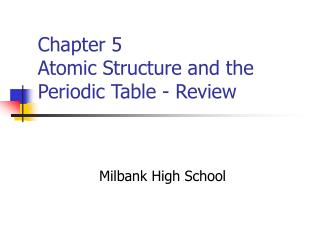DownloadDownload PresentationChapter 5 Atomic Structure and the Periodic Table - Review

# Chapter 5 Atomic Structure and the Periodic Table - Review

Télécharger la présentation## Chapter 5 Atomic Structure and the Periodic Table - Review

- - - - - - - - - - - - - - - - - - - - - - - - - - - E N D - - - - - - - - - - - - - - - - - - - - - - - - - - -
##### Presentation Transcript

1. Chapter 5 Atomic Structure and the Periodic Table - Review Milbank High School

2. Chapter 5 Review • What is the smallest particle of an element that retains the properties of that element? • Who first suggested the idea of atoms, in the 4th century b.c.? • The number of atoms in a copper coin suggests that ...

3. Chapter 5 Review • Know the ideas of Dalton’s atomic theory. • What particles form the nucleus of an atom? • What parts of Dalton’s original atomic theory were revised about a century ago?

4. Chapter 5 Review • Know about the discovery of electrons. • Why did J. J. Thomson reason that electrons must be a part of the atoms of all elements? • What hypothesis led to the discovery of the proton?

5. Chapter 5 Review • Who discovered the neutron, and in what year? • How does the mass of a neutron compare to the mass of a proton? • Dalton theorized that atoms are indivisible, and atoms of the same element are identical. Today ...

6. Chapter 5 Review • As a result of the discovery of the nucleus by Rutherford, what model described an atom? • Describe the nucleus of an atom. • Know the properties of the electron. • Atoms have what charge, and how many protons and electrons?

7. Chapter 5 Review • Describe the number of protons and electrons in a given element, such as In. • The atomic number of an element is the total number of … • An element has an atomic number of 80. How many protons & electrons?

8. Chapter 5 Review • The mass number of an element is equal to … • How are the number of neutrons in the nucleus of an atom calculated? • The sum of the protons and neutrons in an atom equals the ...

9. Chapter 5 Review • Using the periodic table, determine the number of neutrons in 16O. • How many protons, electrons, and neutrons does an atom with an atomic number of 50 and a mass number of 120 contain?

10. Chapter 5 Review • How do the isotopes of hydrogen-1 and hydrogen-2 differ? • What does the number 84 in the name krypton-84 represent? • What unit is used to measure average relative atomic mass?

11. Chapter 5 Review • Different elements have different numbers of … • All atoms of the same element have the same … • Why do chemists use relative comparisons of masses of atoms?

12. Chapter 5 Review • What does one atomic mass unit (a.m.u.) equal? • Isotopes of the same element have different ____ and ____. • What does 23892U represent? • Compare 8038X and 8138X.

13. Chapter 5 Review • What are isotopes? • Compare 2010X and 2110X. • The atomic mass of an element is equal to … • Calculate average atomic mass if one isotope of mass 20 is 25 % abundant, and another has a mass 22 is 75%?

14. Chapter 5 Review • The atomic mass of an element depends on ____, _____, and ___. • What are the Group A elements also known as? • Which is/are a representative element(s)? Fe, Hg, U, Te, or Y

15. Chapter 5 Review • Of the elements Pt, Sc, V, Li, and Kr, which is/are nonmetal(s)? • What is each vertical column of elements in the periodic table called? • Approximately how many elements exist today?

16. Chapter 5 Review • What criterion is used to arrange the elements in rows and columns on the periodic table? • What category (metals, nonmetals, or metalloids) includes the majority of the elements?

17. Chapter 5 Review • A mystery element, Q, is a nonlustrous solid and a poor conductor of electricity. To what category of elements does it belong? • Who first arranged the elements according to nuclear charge?